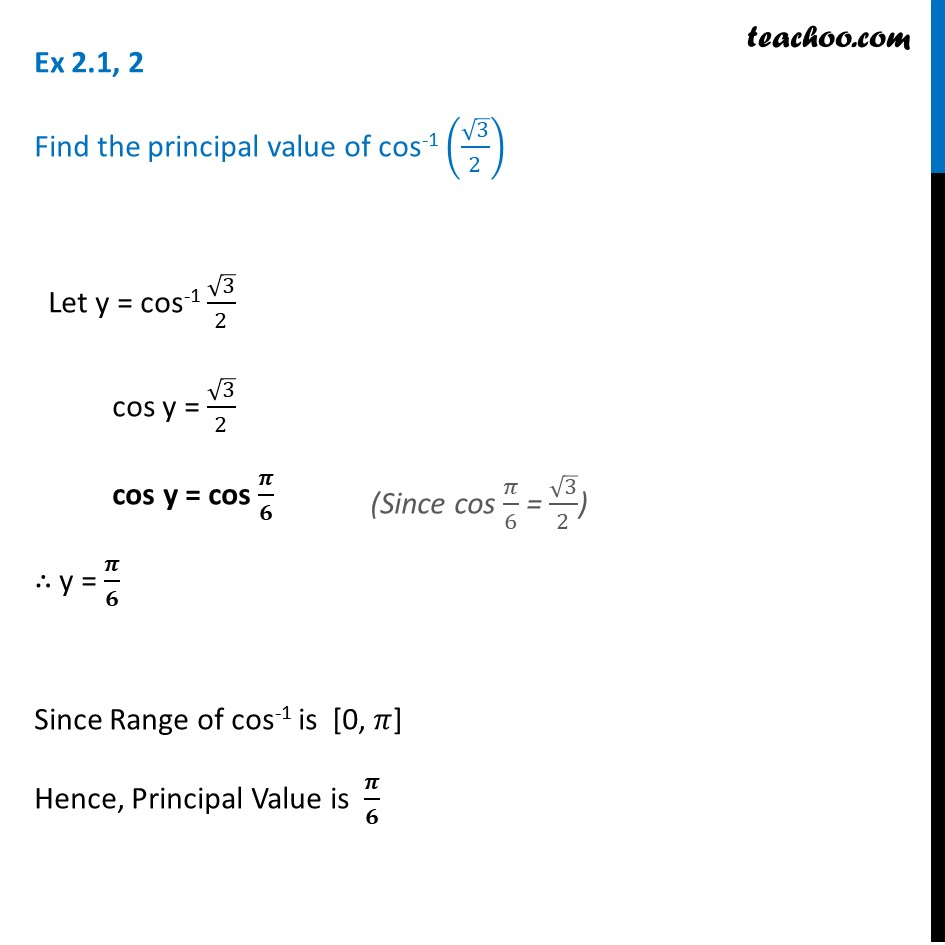1. Chapter 2 Class 12 Inverse Trigonometric Functions (Term 1)
2. Serial order wise
3. Ex 2.1

Transcript

Ex 2.1, 2 Find the principal value of cos-1 (√3/2) Let y = cos-1 √3/2 cos y = √3/2 cos y = cos 𝝅/𝟔 ∴ y = 𝝅/𝟔 Since Range of cos-1 is [0, 𝜋] Hence, Principal Value is 𝝅/𝟔 (Since cos 𝜋/6 = √3/2)